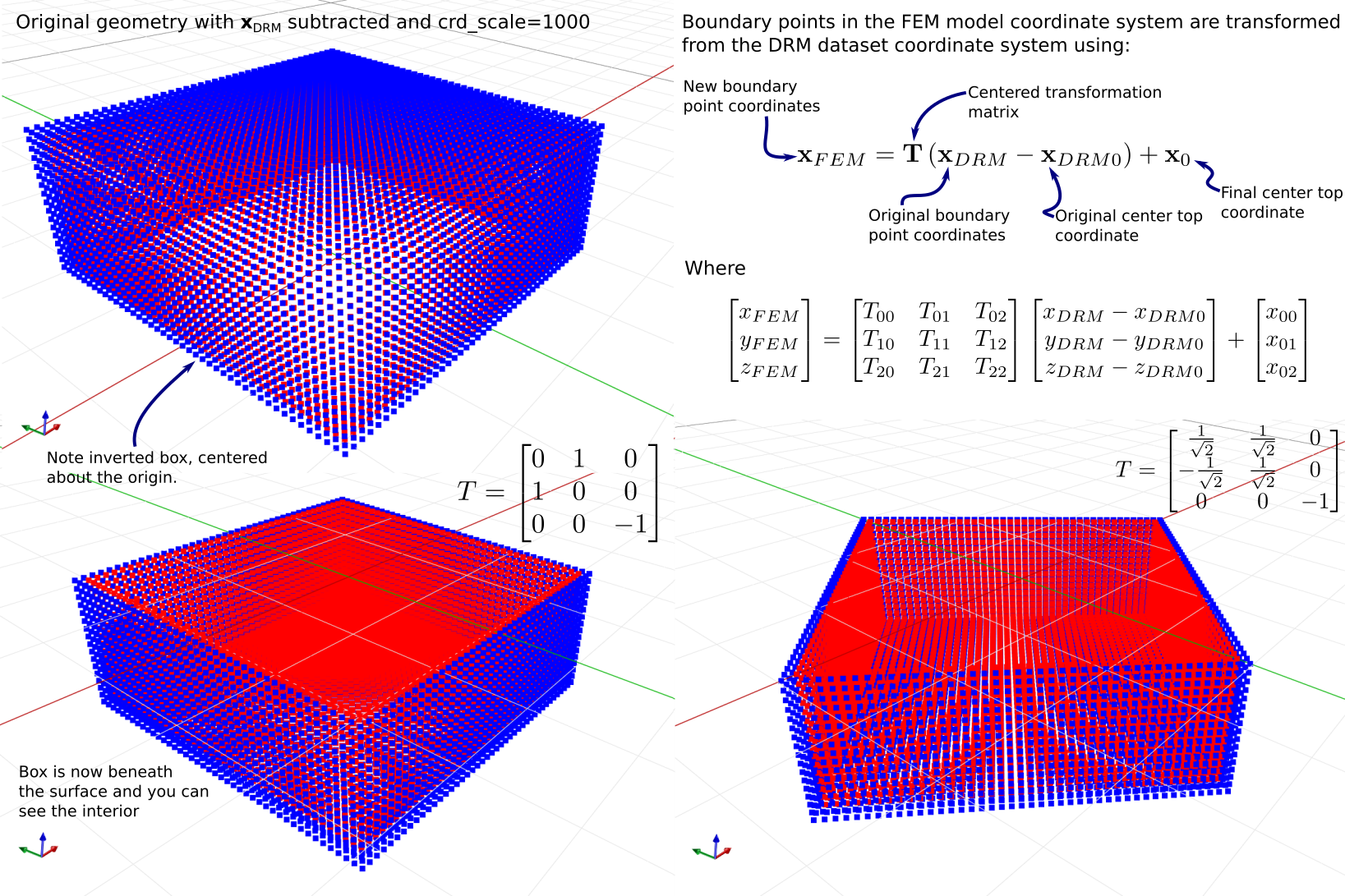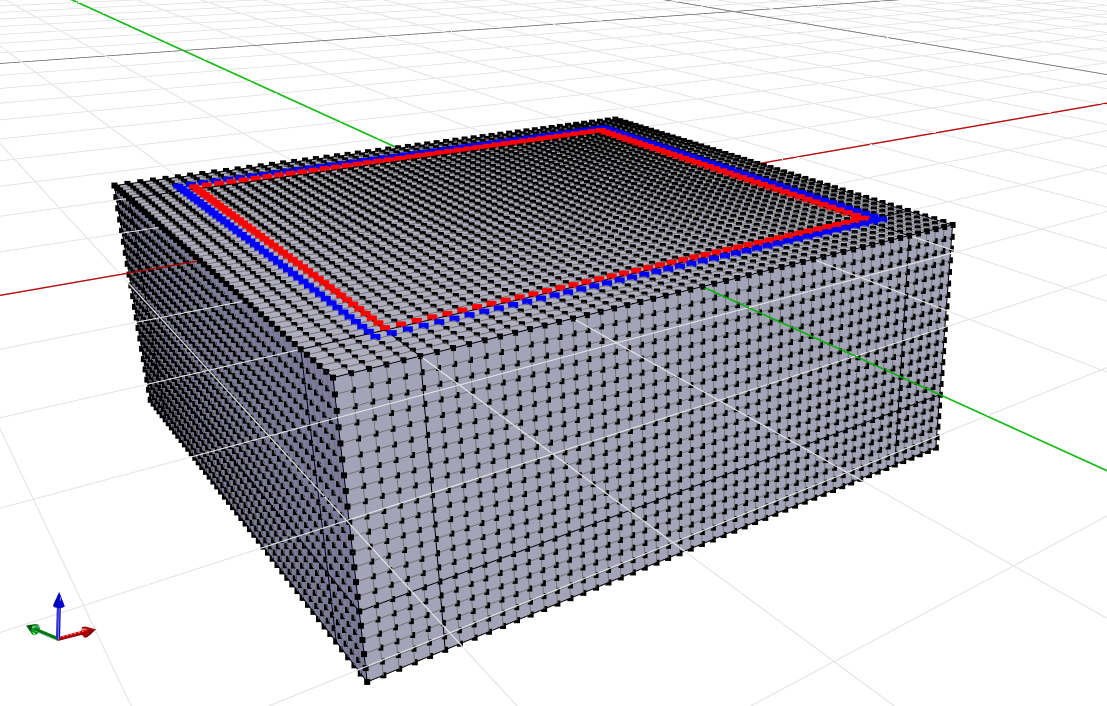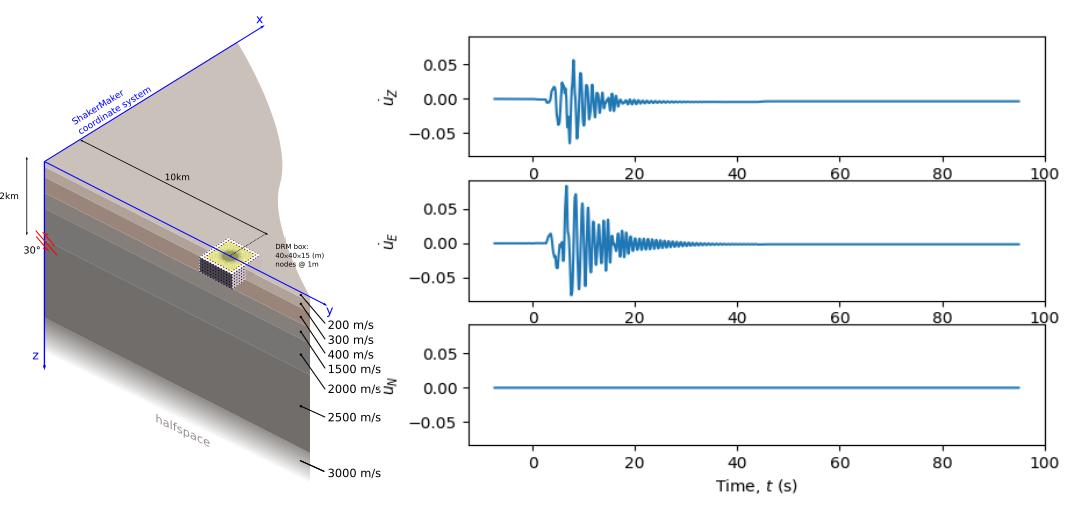# 3.1.11.4. H5DRM Pattern¶

Perform earthquake time-history analysis using the domain reduction method (DRM).

The H5DRM pattern implements the DRM using the H5DRM data format. It directly reads the dataset, performs node matching, identifies the connecting elements, and applies the loads and (linear) interpolation of displacements and accelerations in time.

pattern H5DRM $patternTag "/path/to/H5DRM/dataset"$factor $crd_scale$distance_tolerance $do_coordinate_transformation$T00 $T01$T02 $T10$T11 $T12$T20 $T21$T22 $x00$x01 $x02 All paramters are required at this point! Argument Type Description$factor

float

multiply DRM dataset displacements and accelerations by this value

$crd_scale float multiply (x, y, z) coordinates of dataset points by this value (typically for unit change between dataset and FE model)$distance_tolerance

float

tolerance for DRM point to FE mesh matching. Set to a size similar to the node distance near the DRM boundary.

$do_coordinate_transformation integer =1 apply a coordinate transformation to the (x, y, z) coordinates of dataset points before matching$T00

float

00 component of the transformation matrix

$T01 float 01 component of the transformation matrix$T02

float

02 component of the transformation matrix

$T10 float 10 component of the transformation matrix$T11

float

11 component of the transformation matrix

$T12 float 12 component of the transformation matrix$T20

float

20 component of the transformation matrix

$T21 float 21 component of the transformation matrix$T22

float

22 component of the transformation matrix

$x00 float x location of the top-center node of the DRM Box after transformation$x01

float

y location of the top-center node of the DRM Box after transformation

\$x02

float

z location of the top-center node of the DRM Box after transformation

This load pattern takes input from DRM datasets formatted using the H5DRM data-format specified in , which uses the HDF5 library for IO. These datasets can be produced natively for 1-D crust models by the ShakerMaker python library (it was designed to do this). Otherwise, the HDF5 format on which H5DRM is based is simple enough that output from other seismological software can be easily cast into this format (in python use the h5py library). Hopefully, other programs will provide native support for H5DRM in the future.

Because the H5DRM dataset format is designed to facilitate the sharing of datasets for DRM analysis, the coordinate systems with which the DRM boundary points are represented are generally unrelated to those of the local-scale (OpenSees FEM) model. Thus, we provide a means for transforming between coordinates systems in the implementation of the H5DRM load pattern, which is applied to the DRM boundary point coordinates before the node matching. This transformation is described in the following image.Example: Free field response

In this example, the free-field site response to motions input with DRM are computed. When the simulation conditions used to develop the DRM motion dataset match those of the local-site, then there are (approximately) no out-going waves in the model to be damped. This constitutes a basic check or validation to both the DRM datset and the local-scale FEM model, which is useful to perform before any other analysis with DRM.

Model conditions:• Local site conditions are $$V_s = 200 \frac{m}{s}$$, $$\nu = 0.25$$, $$\rho = 2000 \frac{kg}{m^3}$$ for the top 20m which covers the complete FEM model and DRM dataset.

• Motions generated with ShakerMaker with this python script: drm-example-santiago-1.py?

• DRM motions come from a point source 10km away in the X direction (of the FEM model) and 3 km deep. The point source dips at an angle of 30°, which means that only motions in the X-Z planes occur due to the location of the site. The following is the expected surface response at the site where DRM motions are recorded (in the FEM model X is east, Y is north and Z is up):• The source time-funciton is a Brune source with a corner frequency of $$f_0 = 4 Hz$$.

• The cutoff frequency for the mesh is set to $$f_{max} = 10Hz$$, which results in a required mesh size of $$dx = 1m$$ and a time-step of $$dt = 0.005$$.

• DRM box size is $$40m \times 40m \times 15m$$

• The interior of the DRM box is set to have a Rayleigh-damping of 0.1% while the exterior is set to 20%, this eliminates unresolved frequencies (and out-going waves for non free-field models).

• The OpenSees model was prepared in STKO for single-processor analysis and also multi-processor analysis (OpenSeesMP). You can download the TCL files of the model in any version here:

By looking in analysis-steps.tcl for any of the above examples, you can see the TCL line for the H5DRM load pattern is:

pattern H5DRM 3 "C:/path/jaabe/Documents/DRM-example/santiago1.h5drm" 1.0 1000.0 0.001 1   0.0 1.0 0.0 1.0 -0.0 0.0 0.0 0.0 -1.0   0.0 0.0 0.0


Code Developed by: José Antonio Abell (UANDES). For issues, start a new issue on the OpenSees github repo and tag me (@jaabell).

 Jose A. Abell., Jorge G.F. Crempien, Matías Recabarren ShakerMaker: A framework that simplifies the simulation of seismic ground-motions. SoftwareX. https://doi.org/10.1016/j.softx.2021.100911